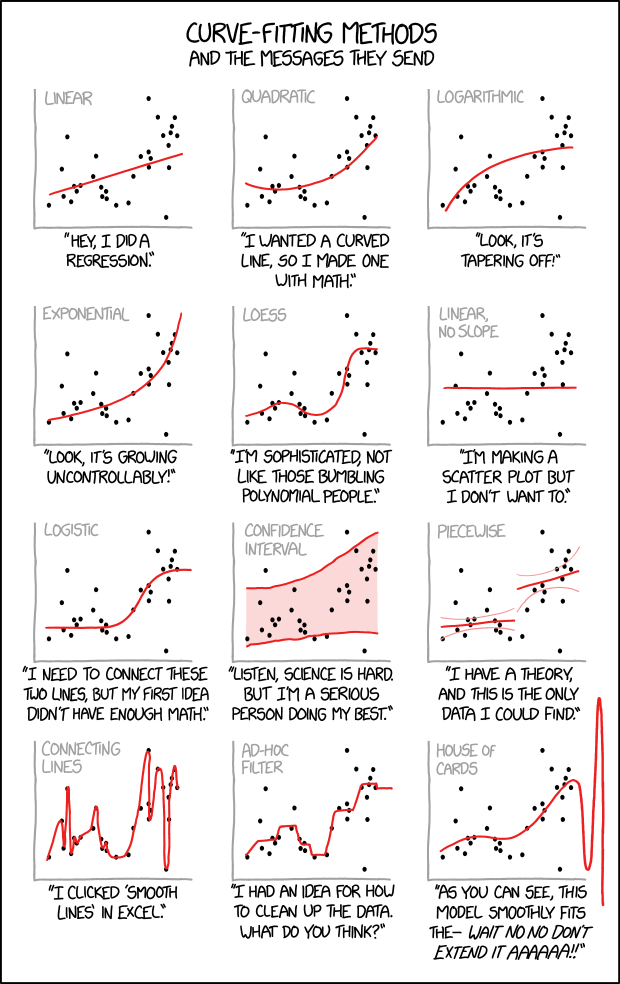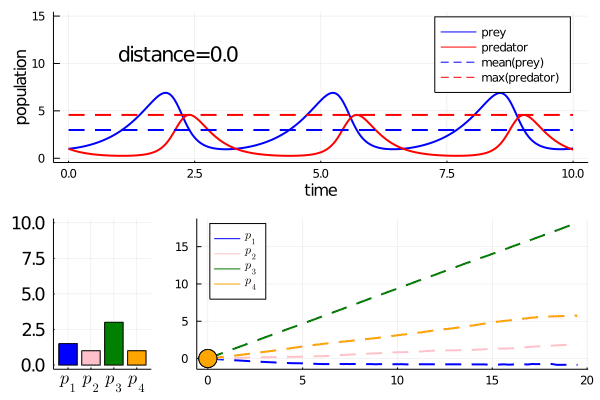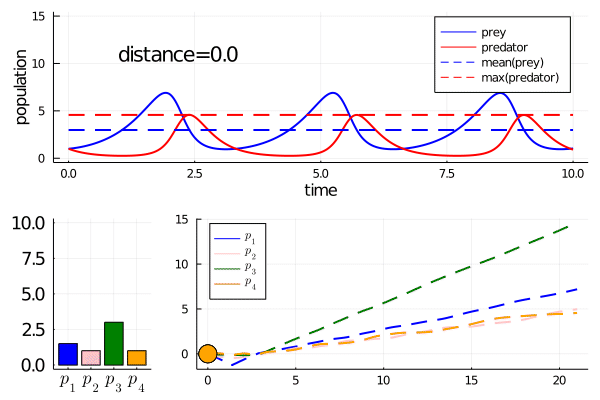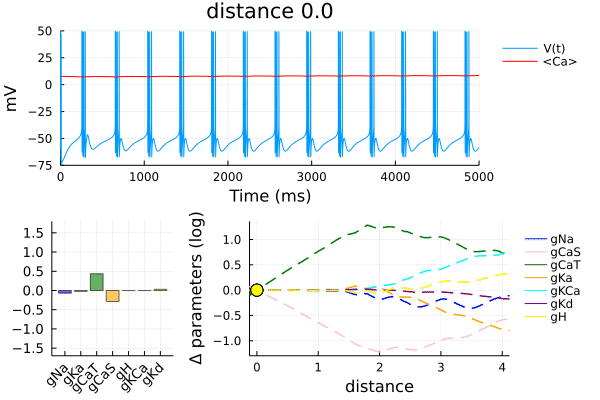# MinimallyDisruptiveCurves.jl

Because building a good model is hardBut extracting useful insight from a model is harder(Credit: https://xkcd.com/793/ and https://xkcd.com/2048/)

## What it does (short form)

At its core, only one thing:

Finds the hidden, potentially nonlinear relationships between model parameters that best preserve user-defined features of model behaviour.

(...by solving a differential equation on the parameters)

## Explanation by example

• This is the four parameter Lotka Volterra differential equation model of predator-prey dynamics. Covered in more detail in Examples

$\frac{d 🐁}{dt} = p_1 🐁 - p_2🐁 🦉 \\ \frac{d 🦉}{dt} = -p_3🦉 + p_4 🐁 🦉$

(🐁 are born at rate $p_1$, die/are eaten at rate $p_2$. 🦉 die at rate $p_3$, are born at rate $p_4$.)

• Model dynamics at $p = [1.5,1.0,3.0,1.0]$:• Let's quantify good model behaviour as having a particular, fixed, value for

• mean prey population over time

• max predator population over time

• Each minimally disruptive curve finds a different way to change parameters while 'minimally disrupting' these model features:

MDC 1MDC 2• Both curves evolved in seconds on my laptop.

• MDC generation was automatic. No human insight required!

• Want more involved examples with more complicated models? Check out the Pluto.jl notebooks on https://github.com/Dhruva2/MDCExamples.git.

• Here is a teaser: parameter relationships on a model of a bursting neuron that preserve it's physiological, time-averaged calcium concentration, and the 'bursting' phenotype of its' voltage trace. Parameters here are the ion-channel densities, which are physiologically variable:## Schematic

Minimally disruptive curve(bracketed blocks are optional. Ideal outputs could be experimental data). Choice of cost/loss function is arbitrary, as long as it's differentiable.

Compared to model fitting/optimisation (which this is not)• You start with an initial set of parameters for which the model 'works' as you want it to (as quantified by a cost/loss function). You can get this from model fitting/optimisation.

• Each minimally disruptive curve is a continuous path in parameter space. Any point on the path is a set of parameters for which the model 'works' (nearly) as well as the initial set of parameters.

• The job of the MD curve generator is to

get as far away as possible from the initial parameters, while keeping the cost function as low as possible.

By the way

• As far away as possible implies some notion of distance (metric) on parameter space. Maybe you're interested in relative, rather than absolute changes. Or you're particularly interested in changes to a few particular parameters. MinimallyDisruptiveCurves.jl makes it easy to make your own custom metric (see Features section).

By the way

Play around with curve specifications such as :

• the initial curve direction

• which parameters the curve can(not) change

• which parameters the curve is biased towards changing

• reparameterising the cost function

... to get different minimally disruptive curves yielding different model insights.

Not happy with the explanation? See the following sources for more information:

SourceRigorous enoughExcessively rigorousExcessively verboseUnreliable
My thesis, ch. 5 (ch. 4 is relevant too)xx
xx
How it worksx
dvr23@cam.ac.ukx

## Use Cases

You have a mathematical model (of any flavour), and you want to answer questions like:

• Which model interactions could I kill without greatly affecting model behaviour?

• Is there a hidden approximation I could make in the model that wouldn't greatly change behaviour?

• Are model parameters structurally / practically identifiable from data? If not, which (functions of the) parameters are unidentifiable?

• (Dually) What spaces of parameters are (approximately/exactly) consistent with the data / desired behaviour?

• Could changes in parameter $x$ could be continuously compensated for by changes in parameters $y$ and $z$? Or by $u$, $v$, and $w$? Or by any parameters at all? If so, what kind of compensatory changes?

By the way

• We provide Examples in which MD curves answer all of the above questions

(on differential equation models from systems biology/neuroscience due to author prejudice. The package is model-agnostic.)

• If you find any other use case, let me know!

## Basic workflowBy the way

• MinimallyDisruptiveCurves never interacts directly with your model. Only the cost function. So it is agnostic to the model / how you coded the model

• Your cost function can be anything, as long as it's differentiable. Quantify how well the model matches data, oscillates at 4Hz or does backflips. Your choice.

What you need

1. A julia function (the cost function) with two methods as shown:

# method 1
function cost(params)
...
return cost
end

# method 2
...
# It must provide the gradient of the cost with respect to parameters.
return cost
end
1. An initial set of parameters, p0, that are locally minimal with respect to the cost function. So

grad_holder = rand(size(p0))
# now grad_holder ≈ zeros(size(grad_holder))
• How do you get such a p0? By fitting the model to the cost function. In Julia, you can choose from a variety of optimisation packages. I use Optim.jl in the examples.

By the way

Calculating the gradients of cost functions of complicated models is hard right?

Not these days! Automatic differentiation of near-arbitrary code is one of the big advantages of Julia. See the Examples. Also note

• The two-method specification of the cost function above is shared with most julia optimisation packages (e.g. Optim.jl). So you can incorporate these seamlessly in a MinimallyDisruptiveCurves.jl workflow, for creating/pre-minimising cost functions.

• DiffEqParamEstim.jl makes cost functions of the required form for differential equation-based models.

• Otherwise, Zygote.jl and ForwardDiff.jl can collectively take the gradient of pretty much any differentiable Julia code between them.

• If all else fails, MinimallyDisruptiveCurves.jl provides a function: make_fd_differentiable(cost), that spits out a cost function of the required form, with a finite-difference step to calculate the gradient. But using finite diffence differentiation with Julia is like using a Ferrari to do the school run.

1. An initial curve direction dp0.

• You can find the minimally disruptive initial direction using local sensitivity analysis. MinimallyDisruptiveCurves.jl has tools to help you with that. Or you can find a custom direction involving a few parameters you are interested in.

(4.) A momentum hyperparameter: mom, with a default value. The curve stops evolving once $C(p) \geq mom$.

Then you can run the following code:

span = (-50.,100.)
# the length of the curve-to-be.
# Negative values => two curves are evolved in parallel, in the directions plus/minus dp0, and with lengths span and span.

eprob = MDCProblem(cost, p0, dp0, mom, span)
# create an object eprob that stores all the curve information

mdc = evolve(eprob, Tsit5)
# solve the differential equation that evolves the md curve.
# using (in this case) the Tsit5 ODE solver. You can use any solver defined in DifferentialEquation.jl.
using Plots
plot(mdc)
# custom plotting of the important features of the MD curve.

Note that mdc::MDCSolution. This type comes with functionality for inspecting/interpolating the curve at different points. All hijacked from DifferentialEquations.jl, of course.

## Features

Code whatever model you like, however you like

• MinimallyDisruptiveCurves.jl does not directly interact with your model. You only need to provide a cost function as described above. So there is no constraint on the model simulation step inside the cost function. You could even have your model simulation in another language, and use e.g. pycall.jl or ccall.jl.

Avoids the curse of dimensionality

• Since this package is not based on sampling parameter space, it doesn't suffer from the curse of dimensionality in models with many parameters.

• Generating a minimally disruptive curve usually requires somewhere between a few hundred, and a few thousand, evaluations of your cost function (and its gradient). That's the major computational cost.

• If you use reverse-mode automatic differentiation to compute your cost gradient, then the computational complexity of gradient evaluation grows favourably with the number of parameters. That's why all these machine learning models with millions of parameters optimise so fast.

• Often you want a model to behave one way for one input, and another way for another input. Each case induces a separate cost function. You can sum cost functions using the function sum_losses(arr), where arr is an array of cost functions. The resulting summed cost function will run each component cost on a separate thread. So you can have a computationally efficient cost function with respect to model output on a library of inputs.

Easily manipulate cost functions

• A lot of MinimallyDisruptiveCurves.jl workflows will require regularly fixing/freeing cost function parameters, or completely reparameterising cost functions.

• E.G. you might be interested in only allowing a few parameters to change. You might be interested in relative, rather than absolute, changes. You might want to bias the curve to align with a particular parameter(s).

• MinimallyDisruptiveCurves.jl allows you to easily build composable TransformationStructure objects, with which you can reparameterise your cost function. These objects define a transformation:

$T: \mathbb{R}^N \to \mathbb{R}^M$

from the 'old' $N$-dimensional parameter space to the 'new' $M$-dimensional space. You just need to define $T$ and $T^{-1}$ to make the object. You can then use the function:

C_new = transform_cost(C_old, p0, tr::TransformationStructure; kwargs)

to get a new, differentiable cost function $\tilde{C}$ satisfying

$\tilde{C}(T(p)) = C(p).$
• MinimallyDisruptiveCurves.jl comes preloaded with some useful TransformationStructures such as

• $T = log \circ abs$ if you're interested in relative changes to parameters.

• $T: p_i -> k*p_i$ for your choice of $k$, $i$ if you're interested in biasing the curve to (not) align with particular parameters.

• Fixing/freeing (all except / ) named parameters.

• But the source code for each of these preloaded TransformationStructures is $\leq$ 10 lines, so you really can just make your own.

## Caveats

Your cost function needs to be differentiable.

• Your cost function is a mathematical expression of what you want your model to do. A little bit of ingenuity can usually find differentiable cost functions that successfully express what you want the model to do.

• Otherwise, switch your brain off and take your cost as the L2 deviation from some ideal model output.

• Or finite difference the gradient with make_fd_differentiable(cost), close your eyes, and hope for the best.

MinimallyDisruptiveCurves.jl generates 1d functional relationships (i.e.: curves)

There may be higher-dimensional functional relationships (i.e. surfaces or hyper-surfaces) in parameter space, over which model behaviour is approximately preserved. You won't automatically find these using MinimallyDisruptiveCurves.jl. But by evolving multiple curves with different initial conditions / at different points in parameter space, you could get somewhere.

Not convinced this is the right way to do things? has a literature review of other methods, and the relative advantages/disadvantages. I won't rewrite it here.

## Citing, Acknowledgements

The algorithm: (minus a few tweaks)

@article{raman2017delineating,
title={Delineating parameter unidentifiabilities in complex models},
author={Raman, Dhruva V and Anderson, James and Papachristodoulou, Antonis},
journal={Physical Review E},
volume={95},
number={3},
pages={032314},
year={2017},
publisher={APS}
}

The software: as yet unpublished, keep your eye on this!

This work was supported by European Research Council grant StG 2016 FLEXNEURO (716643). Thanks!